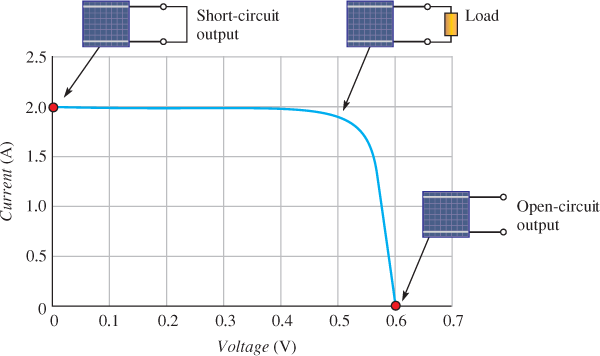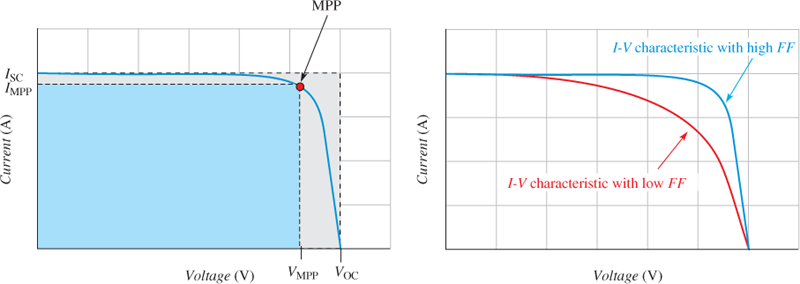Home / Renewable Energy / Photovoltaic (PV) Cell: Characteristics and Parameters

Photovoltaic (PV) Cell: Characteristics and Parameters

Want create site? Find Free WordPress Themes and plugins.

PV cell characterization involves measuring the cell’s electrical performance characteristics to determine conversion efficiency and critical parameters. The conversion efficiency is a measure of how much incident light energy is converted into electrical energy.

The optimum operating point for maximum output power is also a critical parameter, as is a spectral response. That is, how the cell responds to various light frequencies.

Other important characteristics include how the current varies as a function of the output voltage and as a function of light intensity or irradiance.

PV Cell Current-Voltage (I-V) Curves

The current-voltage (I-V) curve for a PV cell shows that the current is essentially constant over a range of output voltages for a specified amount of incident light energy.Figure 1: Typical I-V Characteristic Curve for a PV Cell

Figure 1 shows a typical I-V curve for which the short-circuit output current, ISC is 2 A. Because the output terminals are shorted, the output voltage is 0 V. For an open output, the voltage, VOC is maximum (0.6 V) in this case, but the current is 0 A, as indicated.

PV Cell Output Power

The output power of the PV cell is voltage times current, so there is no output power for a short-circuit condition because of VOUT or for an open-circuit condition because of IOUT = 0.

Above the short-circuit point, the PV cell operates with a resistive load.

Between the short-circuit point and the knee of the curve, the output power depends on the voltage because the current is essentially constant.

The maximum output power occurs in the knee at the point, called the maximum power point (MPP), where the voltage is approximately 0.52 V. After that point, the curve decreases drastically and the output power declines, as illustrated in Figure 2. In the figure, the blue curve is the power. In this particular case, the maximum output power is POUT (MAX) = VMPPTIMPP = (0.52 V) (1.85 A) = 0.96 W.

For maximum power, the load on the cell would have to be:

${{R}_{L}}=\frac{{{V}_{MPP}}}{{{I}_{MPP}}}=\frac{0.52}{1.85}=0.281\Omega$

The output voltage of a PV cell is affected only slightly by the amount of light intensity (irradiance), but the current, and thus the power, decreases as the irradiance decreases.

PV cell parameters are usually specified under standard test conditions (STC) at a total irradiance of 1 sun (1,000 W/m2), a temperature of 25°C and coefficient of air mass (AM) of 1.5. The AM is the path length of solar radiation relative to the path length at zenith at sea level.

The AM at zenith at sea level is 1. Figure 3 shows typical I-V curves for three values of irradiance. Cells vary in the amount of current at a given irradiance.

Energy Conversion Efficiency

The efficiency of a PV cell is the ratio of light energy falling on the cell to the light energy that is converted into electrical energy.

It is expressed as a percentage, as shown in the following formula:

$Efficiency=\frac{{{P}_{out(max)}}}{E\times A}\times 100$

Where

Pout (max) is the maximum electrical power output of the cell, in watts (W)

E is the irradiance (light energy) at the surface of the cell, in watts/meter2 (W/m2)

A is the surface area of the cell, in meter2 (m2)Figure 2: Power Curve for a Typical PV CellFigure 3: I-V Characteristics as a Function of Irradiance

PV cells are typically square, with sides ranging from about 10 mm (0.3937 inches) to 127 mm (5 inches) or more on a side. Typical efficiencies range from 14% to 18% for a monocrystalline silicon PV cell. Some manufacturers claim efficiencies greater than 18%.

Factors That Effect Conversion Efficiency

Several factors determine the efficiency of a PV cell: the type of cell, the reflectance efficiency of the cell’s surface, the thermodynamic efficiency limit, the quantum efficiency, the maximum power point, and internal resistances.

When light photons strike the PV cell, some are reflected and some are absorbed. The non-reflective cell coating minimizes the percentage of photons that are reflected. Certain types of irregular cell surfaces help to decrease the reflection of photons.

The angle of incidence of the photons striking the cell surface is also a factor in determining the amount of reflection.

The thermodynamic efficiency relates to the photon energy. Photons with energies below the band-gap energy of silicon (1.12 eV) cannot produce electron-hole pairs, and their energy is converted to heat rather than to an electrical output.

Photons with energies at or above the bandgap create electron-hole pairs and contribute to the electrical output. If the photon energy is greater than the band-gap energy, the excess is transferred to the electron-hole pair and eventually dissipated.

Quantum efficiency relates to the number of absorbed photons that contribute to the electrical current for a short-circuit condition.

Not all of the electrons that are freed from an electron-hole pair end up as current. Some electrons lose energy and recombine with a hole. Quantum efficiency depends on the wavelength of the light because some wavelengths are absorbed more efficiently than others.

Finally, the operation of the Photovoltaic (PV) cell at its maximum power point is vital to the conversion efficiency. As you have seen, the maximum power point occurs in the knee of the I-V characteristic curve as determined by the load.

In solar power systems, a method called Maximum Power Point Tracking (MPPT) is used to maintain maximum output power.

The power output of solar modules can be boosted by 10% just by applying a large transparent sticker to the front. The sticker is a polymer film embossed with microstructures that bend incoming sunlight. The result is that the active materials in the panels absorb more light and convert more of it into electricity.

PV Cell Fill Factor

The fill factor of a PV cell is an important parameter in evaluating its performance because it provides a measure of how close a PV cell comes to providing its maximum theoretical output power.

The fill factor (FF) is the ratio of the cell’s actual maximum power output (VMPP× IMPP) to its theoretical power output (VOC × ISC).

$FF={}^{\left( {{V}_{MPP}} \right)\left( {{I}_{MPP}} \right)}/{}_{\left( {{V}_{OC}} \right)\left( {{I}_{SC}} \right)}$Figure 4 Fill Factor for a PV Cell

Where

FF is the fill factor (dimensionless)

VMPP is the voltage at the maximum power point, in volts (V)

IMPP is the current at the maximum power point, in amperes (A)

VOC is the open circuit voltage, in volts (V)

ISC is the short circuit current, in amperes (A)

Typical commercial solar cells have a fill factor greater than 0.7. During the manufacture of commercial solar modules, each PV cell is tested for its fill factor. If the fill factor is low (below 0.7), the cells are considered as lower grade. Figure 4 illustrates the fill factor.

Temperature Dependence of PV Cells

The output voltage and current of a PV cell is temperature dependent. Figure 5 shows that, for a constant light intensity, the open circuit output voltage decreases as the temperature increases (due to a change in the band gap) but the current is affected only by a small amount. The important point here is that a PV cell performs better in cooler temperatures.Figure 5 Effect of Temperature on Output Voltage and Current for a Fixed Light Intensity in a PV Cell

Review Questions

1. What is the voltage at ISC in a PV cell?
2. At what approximate point on the I-V curve does the maximum output power occur?
3. For a photon to be effective in creating electron-hole pairs in a PV cell, how much energy must it have?
4. What factors affect the efficiency of a PV cell?
5. Does the performance of a PV cell get better or worse when the temperature is cooler?
6. What is the fill factor?

1. 0 V
2. 0.53 V
3. Energy must be at or above the band-gap energy.
4. Factors that affect efficiency are the type of cell, the reflectance efficiency of the cell’s surface, the thermodynamic efficiency limit, the quantum efficiency, the maximum power point, and internal resistance.
5. Better
6. Fill factor is the ratio of the cell’s actual maximum power output (VMPP × IMPP) to its theoretical power output (VOC × ISC).
Did you find apk for android? You can find new Free Android Games and apps.